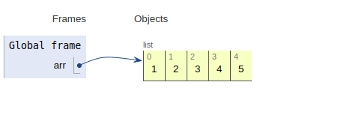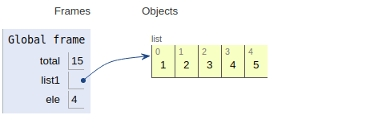# Python program to find sum of elements in list

In this article, we will learn about the solution and approach to solve the given problem statement.

## Problem statement

Given an list as an input, we need to compute the sum of the given list.

Here we have two approaches to consider i.e. using built-in function & using the brute-force approach.

## Approach 1 − Using built-in function

### Example

Live Demo

# main
arr = [1,2,3,4,5]
ans = sum(arr)
print ('Sum of the array is ',ans)

### Output

15

All the variables & functions are declared in the global scope and are shown below.## Approach 2 − Using brute-force method

### Example

Live Demo

total = 0
# creating a list
list1 =[1,2,3,4,5]
for ele in range(0, len(list1)):
total = total + list1[ele]
# main
print("Sum of all elements in given list: ", total)

### Output

15

All the variables & functions are declared in the global scope and are shown below.